# Using the rerddapXtracto routines

## Introduction

`rerddapXtracto` is an R package developed to subset and extract satellite and other oceanographic related data from any ERDDAP server using the R package rerddap developed by Scott Chamberlain and the wonderful people at rOpenSci. ERDDAP is a simple to use yet powerful web data service developed by Bob Simons. `rerddapXtracto` extends the `rerddap` package by being able to extract data for a moving point in time along a user-supplied set of longitude, latitude and time points; and also extracting data within a polygon (through time). `rerddapXtracto` extends the functionality of the R package xtractomatic by being able to work with (hopefully) most gridded datasets available from any ERDDAP server. The disadvantage compared to `xtractomatic` is that the user has to do more work to obtain information about the dataset to be accessed, while in `xtractomatic` that information is built in.

This version has several major changes. In both `rxtracto()` and `rxtracto_3D()` the zcoord is not limited to be at a set location. That means for `rxtracto_3D()` that if the zCoord needs to be given for any reason, then it must be of length two, and for `rxtracto()` if the zCoord needs to be given for any reason, it must be of the same length as the other coordinates, and can also have a “zlen”“, like”xlen" and “ylen”, that defines a bounding box within which to make the extract. The advantage of this is it allows `rxtracto()` to make extracts moving in (x, y, z, t) space.

Second there are now two functions, `plotTrack()` for tracks and `plotBBox()` for grids, that produce quick maps of the output using the R package `plotdap`. All of the examples have been changed to use these functions. Bounding box extracts, as in `rxtracto_3D()`, can be done just using `rerddap`, but the `rerddap` function `griddap()` returns a “melted” version of the data, while `rxtracto_3D()` retains the grid structure. Also, `rxtractogon()` uses `rxtracto_3D()` and therefore `plotBBox()` can be used to plot the the results from `rxtractogon()`. These functions should also work with the output from the R package `xtractomatic`. For when more control of plot is desired several examples uses `ggplot2` directly.

Third, it is now possible to make an extract that crosses the dateline for a dataset that is on a (-180, 180) longitude grid. This must be used with care, because some of the built-in checks are disabled for this case, and the requests must have longitudes on a (0, 360) longitude grid else errors will occur. This is particularly important for `rxtracto()` where the observed longitude point may not cross the dateline but the bounding box defined by “xlen” may cross it.

Finally, the plotting packages now allow for user defined continental outlines (important if crossing the dateline), a user defined crs, as well as animation.

### The Main xtractomatic functions

There are three main data extraction functions in the `rerddapXtracto` package:

• `rxtracto <- function(dataInfo, parameter = NULL, xcoord = NULL, ycoord = NULL, zcoord = NULL, tcoord = NULL, xlen = 0., ylen = 0., zlen = 0., xName = 'longitude', yName = 'latitude', zName = 'altitude', tName = 'time', verbose = FALSE, progress_bar = FALSE)`

• `rxtracto_3D <- function(dataInfo, parameter = NULL, xcoord = NULL, ycoord = NULL, zcoord = NULL, tcoord = NULL, xName = 'longitude', yName = 'latitude', zName = 'altitude', tName = 'time', verbose = FALSE, cache_remove = TRUE)`

• `rxtractogon <- function(dataInfo, parameter, xcoord = NULL, ycoord = NULL, zcoord = NULL, tcoord = NULL, xName = 'longitude', yName = 'latitude', zName = 'altitude', tName = 'time', verbose = FALSE)`

and two functions for producing maps:

• `plotTrack <- function(resp, xcoord, ycoord, tcoord, plotColor = 'viridis', myFunc = NA, mapData = NULL, crs = NULL, animate = FALSE, cumulative = FALSE, name = NA, shape = 20, size = .5)`

• `plotBBox <- function(resp, plotColor = 'viridis', time = NA, myFunc = NA, mapData = NULL, crs = NULL, animate = FALSE, cumulative = FALSE, name = NA, maxpixels = 10000)`

The data extraction functions are similar to but not identical to the functions in `xtractomatic`. The main differences are having to obtain information about the dataset first using the function `rerddap::info()`, and possibly having to give the names of the coordinate variables, as these can’t be assumed (for example the zcoord could be in sigma coordinates). More specifically:

• dataInfo: the return from an `rerddap::info()` call to a dataset on an ERDDAP server
• parameter: character string containing the name of the parameter to extract
• xName: character string with name of the xcoord in the ERDDAP dataset (default “longitude”)
• yName: character string with name of the ycoord in the ERDDAP dataset (default “latitude”)
• zName: character string with name of the zcoord in the ERDDAP dataset (default “altitude”)
• tName: character string with name of the tcoord in the ERDDAP dataset (default “time”)
• verbose: logical variable (default FALSE) if the the URL request should be verbose

### Time has come today

With all due respect to the ‘Chambers Brothers (their song ’Time Has Come Today’), since any ERDDAP served gridded data can be accessed, a lot more care must be used with the values of “time” passed to `rerddapXtracto` as compared with `xtractomatic`. This is because datasets can have time increments of less than a day, an example of which is given below. ERDDAP maps all times to “Zulu” time, of the form “2016-11-01T00:00:00Z”. The date-time “2016-11-01” gets mapped to “2016-11-01T00:00:00Z”. When passing time limits of resolution finer than a day, be certain to take into account time offsets, and to pass the times in the form above. Times are parsed using the `parse_date()` function of the parsedate package, if in doubt you can use that function to see how the times you are passing will be interpreted.

## Setting up

`rerddapXtracto` uses the R packages `ncdf4`, `parsedate`, `plotdap`, `rerddap`, and `sp`, and these packages (and the packages imported by these packages) must be installed first or `rerddapXtracto` will fail to install.

``````install.packages("ncdf4", dependencies = TRUE)
install.packages("parsedate", dependencies = TRUE)
install.packages("plotdap", dependencies = TRUE)
install.packages("rerddap", dependencies = TRUE)
install.packages("sp", dependencies = TRUE)``````

The `rerddapXtracto` package is available through CRAN and can be installed by:

``install.packages("rerddapXtracto", dependencies = TRUE)``

The development version of the `rerddapXtracto` package is available from Github. To install the development version,

``````install.packages("devtools")
devtools::install_github("rmendels/rerddapXtracto")``````

Note that `plotdap` depends on a number of packages that must be installed. These include the packages `ggplot2`, `raster` and `sf`. To use the animation features, `gganimate` must be installed.

If the other R libraries have been installed they will be found and do not need to be explicitly loaded.

### Using the R code examples

Once installed, to use `rerddapXtracto`:

``library("rerddapXtracto")``

and to use the plotting functions:

``````library("gganimate")
library("ggplot2")
library("plotdap")``````

## Getting Started

The plotting functions are new, and there are some fine points that need to be understood if they are to be used properly, in particular `plotBBox()`. Both `plotTrack()` and `plotBBox()` rearrange the output so that the functions `plotdap::add_tabledap()` and `plotdap::add_griddap()` think that the output is from `rerddap`, and then make the appropriate `plotdap` call. When the data that are passed to `add_griddap()` has multiple time periods, there are two options. The first option is to set the parameter “time” to a function that reduces the data to one dimension in the time coordinate (such as the mean), or else to set “time” equal to “identity” and set “animate” to be “TRUE” which will produce a time animation of the results. If an animation is requested and the option “cumulative” is set to be “TRUE”, then the animation will be cumulative. This is a nice feature for displaying tracks through time. The function `plotBBox()` works the same way, except that the default function is `mean(na.rm = TRUE)`. The following link to examples that show how to use different features of the plotting functions:

• Setting the color palette shows how to use the “plotColor” option. The “plotColor” parameter can be the name of any of the colors included in the `cmocean` package. The `cmocean` colormaps were designed by Kristen Thyng (see https://matplotlib.org/cmocean/ and https://github.com/matplotlib/cmocean), and were initially developed for Python)

• Plot one time period example shows how to manipulate an existing output from `rxtracto_3D()` or `rextractogon()` to plot just one time period.

• Transform the data example shows how to use the “myFunc” option to transform the data before plotting. The function has to be a function of a single argument. This example also shows how to use the “name” option to change the name displayed on the color bar. In this example, we want depth to go downwards in the colorbar, and the name given changed from “altitude”, which is the name on ERDDAP, to the name “Depth”.

• Name example shows how to change the name on the colorbar.

• Modify the graph shows how to use the `plotdap` function `add_ggplot()` to modify a graph once it has been generated.

• Animate a track shows how to animate a track over time.

• Animate a grid shows how to animate a grid with multiple time periods.

The first several examples reproduce some of the examples in the `xtractomatic` vignette, hopefully to make clear how the functions in the two packages relate. One change from the `xtractomatic` vignette is that the plots use the `cmocean` colormaps described above.

The first step is to find the necessary information from the ERDDAP server being used. These include the

In order for `rerddapXtracto` to have this information, as well as the coordinate variables and their limits, and the parameter names, a call must be made to the function `rerddap::info()` for the appropriate datasetID and baseURL:

``````require("rerddap")
## base URL does not need to given because it is the default one
dataInfo <- info('erdMBchla1day')
dataInfo
#> <ERDDAP info> erdMBchla1day
#>  Base URL: https://upwell.pfeg.noaa.gov/erddap/
#>  Dimensions (range):
#>      time: (2006-01-01T12:00:00Z, 2020-06-11T12:00:00Z)
#>      altitude: (0.0, 0.0)
#>      latitude: (-45.0, 65.0)
#>      longitude: (120.0, 320.0)
#>  Variables:
#>      chlorophyll:
#>          Units: mg m-3``````

### An `rxtracto` example

In this section we extract data along a trackline found in the Marlintag38606 dataset, which is the track of a tagged marlin in the Pacific Ocean (courtesy of Dr. Mike Musyl of the Pelagic Research Group LLC), and show some simple plots of the extracted data. Since it cna be a long extract, a progress bar is displayed:

``````require("rerddap")
require("rerddapXtracto")

# First we will copy the Marlintag38606 data into a variable
# called tagData  so that subsequent code will be more generic.

tagData <- Marlintag38606
xpos <- tagData\$lon
ypos <- tagData\$lat
tpos <- tagData\$date
zpos <- rep(0., length(xpos))
swchlInfo <- rerddap::info('erdSWchla8day')
swchl1 <- rxtracto(swchlInfo, parameter = 'chlorophyll', xcoord = xpos, ycoord = ypos, tcoord = tpos, zcoord = zpos, xlen = .2, ylen = .2, progress_bar = TRUE)``````

Note the differences from the `xtractomatic` example.

• the user has to find the datasetID from the given ERDDAP server, in this case ‘erdSWchla8day’ (from https://upwell.pfeg.noaa.gov/erddap/griddap/erdSWchla8day.html)
• the user has to call `rerddap::info('erdSWchla8day')` to obtain information about that dataset
• the user has to know the parameter name desired for that datasetID, in this case ‘chlorophyll’
• the zcoord has to be explicitly given, because ‘erdSWchla8day’ is a 4-D dataset (altitude is a dimension).

### Plotting the results

We plot the track line with the locations colored according to the mean of the satellite `chlorophyll` around that point. Positions where there was a tag location but no `chlorophyll` values are also shown. This example shows the use of the “plotColor” parameter to use the “chlorophyll” color palette.

``````require("ggplot2")
require("plotdap")

myPlot <- plotTrack(swchl1, xpos, ypos, tpos, plotColor = 'algae')
myPlot``````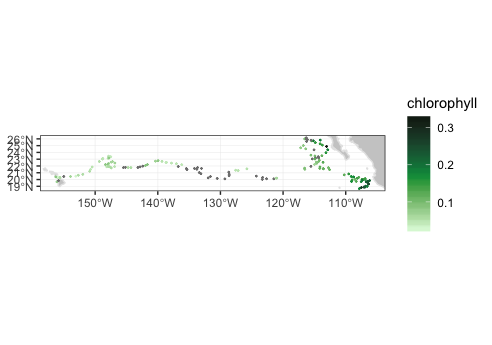### Animating the track

To make a cumulative animation of the track:

``````myPlot <- plotTrack(swchl1, xpos, ypos, tpos, plotColor = 'algae',
animate = TRUE, cumulative = TRUE)``````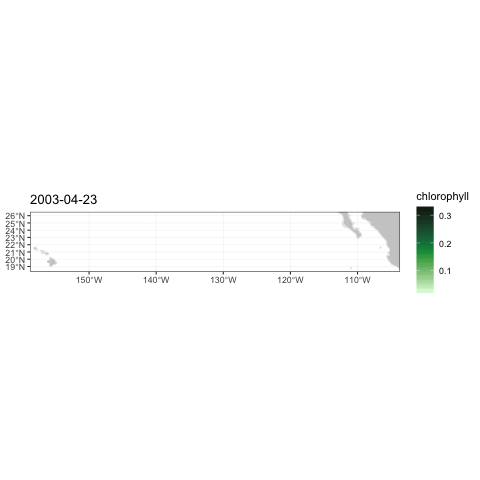marlin Track Animation

### Topography data

The second example from the `xtractomatic` vignette is accessing topographic data along the Marlin track. This example also shows how to pass a function to `plotTrack` to transform the data before plotting, how to change the name shown on the colorbar, and how to call `plotTrack()` if the dataset does not have a time coordinate.

``````require("ggplot2")
require("plotdap")
require("rerddap")
require("rerddapXtracto")
ylim <- c(15, 30)
xlim <- c(-160, -105)
topoInfo <- rerddap::info('etopo360')
topo <- rxtracto(topoInfo, parameter = 'altitude', xcoord = xpos, ycoord = ypos, xlen = .1, ylen = .1)
myFunc = function(x) -x
topoPlot <- plotTrack(topo, xpos, ypos, NA, plotColor = 'dense', name = 'Depth', myFunc = myFunc)
topoPlot``````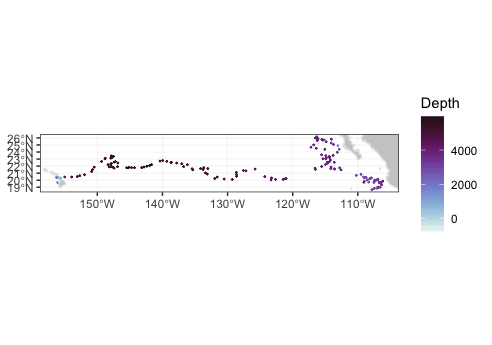Again, note the differences from the `xtractomatic` example:

• the user has to find the datasetID from the given ERDDAP server, in this case ‘etopo360’ (from https://upwell.pfeg.noaa.gov/erddap/griddap/etopo360.html)
• the user has to call `rerddap::info('etopo360')` to obtain information about that dataset
• the user has to know the parameter name desired for that datasetID, in this case ‘altitude’
• only the xcoord and ycoord values need to be given, as ‘etopo360’ is a 2-D dataset, and this is handled more gracefully then in `xtractomatic`.

### Track moving in (x, y, z, t) space.

The following is an artificial example showing a track moving in (x, y, z, t) space. Since the times of the model output change, the actual times are retrieved, and the last three times used in the example.

``````require("rerddap")
urlBase <- "https://erddap.marine.ie/erddap/"
parameter <- "Sea_water_temperature"
dataInfo <- rerddap::info("IMI_CONN_3D", url = urlBase)
#get the actual last 3 times,  and extract from data frame
sstTimes <- dataInfo1[]
sstLats <- c(53.505758092414446, 53.509303546859805, 53.51284900130517)
sstLons <- c(-10.25975390624996, -10.247847656249961, -10.23594140624996)
sstDepths <- c(2, 6, 10)
sstTrack <- rxtracto(dataInfo, parameter = parameter, xcoord = sstLons, ycoord = sstLats, tcoord = sstTimes, zcoord = sstDepths, xlen = .05, ylen = .05, zlen = 0., zName = 'altitude')
#> Registered S3 method overwritten by 'httr':
#>   method           from
#>   print.cache_info hoardr
str(sstTrack)
#> List of 13
#>  \$ mean Sea_water_temperature  : num [1:3] 8.98 9.19 9.77
#>  \$ stdev Sea_water_temperature : num [1:3] 0.15 0.26 0.257
#>  \$ n                           : int [1:3] 493 491 484
#>  \$ satellite date              : chr [1:3] "2020-05-13T22:00:00Z" "2020-05-13T23:00:00Z" "2020-05-14T00:00:00Z"
#>  \$ requested lon min           : num [1:3] -10.3 -10.3 -10.3
#>  \$ requested lon max           : num [1:3] -10.2 -10.2 -10.2
#>  \$ requested lat min           : num [1:3] 53.5 53.5 53.5
#>  \$ requested lat max           : num [1:3] 53.5 53.5 53.5
#>  \$ requested z min             : num [1:3] 2 6 10
#>  \$ requested z max             : num [1:3] 2 6 10
#>  \$ requested date              : chr [1:3] "2020-05-13T22:00:00Z" "2020-05-13T23:00:00Z" "2020-05-14T00:00:00Z"
#>  \$ median Sea_water_temperature: num [1:3] 8.91 9.13 9.74
#>  \$ mad Sea_water_temperature   : num [1:3] 0.0298 0.1713 0.3274
#>  - attr(*, "row.names")= chr [1:3] "1" "2" "3"
#>  - attr(*, "class")= chr [1:2] "list" "rxtractoTrack"``````

### Crossing the dateline

The following is an artificial example of a track that crosses the date-line, using the MUR(Multi-scale Ultra-high Resolution) SST analysis:

``````dataInfo <- rerddap::info('jplMURSST41mday')
parameter <- 'sst'
xcoord <- c(179.7, 179.8, 179.9, 180., 180.1, 180.2, 180.3, 180.4)
ycoord <- c(40, 40, 40, 40, 40, 40, 40, 40)
tcoord <- c('2018-03-16', '2018-03-16', '2018-03-16','2018-03-16','2018-03-16','2018-03-16','2018-03-16','2018-03-16')
xlen <- .05
ylen <- .05
extract <- rxtracto(dataInfo, parameter = parameter, xcoord = xcoord,
ycoord = ycoord, tcoord = tcoord,
xlen = xlen, ylen = ylen)
str(extract)
#> List of 13
#>  \$ mean sst         : num [1:8] 11.1 11.1 11.1 11.2 11.1 ...
#>  \$ stdev sst        : num [1:8] 0.01328 0.00713 0.01025 0.01036 0.01446 ...
#>  \$ n                : int [1:8] 30 35 35 30 30 30 35 35
#>  \$ satellite date   : chr [1:8] "2018-03-16T00:00:00Z" "2018-03-16T00:00:00Z" "2018-03-16T00:00:00Z" "2018-03-16T00:00:00Z" ...
#>  \$ requested lon min: num [1:8] 180 180 180 180 180 ...
#>  \$ requested lon max: num [1:8] 180 180 180 180 180 ...
#>  \$ requested lat min: num [1:8] 40 40 40 40 40 ...
#>  \$ requested lat max: num [1:8] 40 40 40 40 40 ...
#>  \$ requested z min  : logi [1:8] NA NA NA NA NA NA ...
#>  \$ requested z max  : logi [1:8] NA NA NA NA NA NA ...
#>  \$ requested date   : chr [1:8] "2018-03-16" "2018-03-16" "2018-03-16" "2018-03-16" ...
#>  \$ median sst       : num [1:8] 11.1 11.1 11.1 11.2 11.1 ...
#>  \$ mad sst          : num [1:8] 0.01627 0.00525 0.01149 0.01079 0.01744 ...
#>  - attr(*, "row.names")= chr [1:8] "1" "2" "3" "4" ...
#>  - attr(*, "class")= chr [1:2] "list" "rxtractoTrack"``````

## Using `rxtracto_3D`

The function `rxtracto_3D()` adds no new capabilities to `rerddap`, but it does return the data in a structure consistent with the `xtractomatic` function `xtracto_3D()`, is used in the function `rxtractogon()`, and provides a consistent parallel to the functions in the `xtractomatic` package. It also changes latitudes and longitudes to agree with those of the source dataset, and returns a structure where these are mapped back to the request.

### Obtaining VIIRS chlorophyll data

We examine VIIRS chlorophyll for the “latest” data as of when the vignette was generated:

``````require("rerddap")
require("rerddapXtracto")

xpos <- c(-125, -120)
ypos <- c(39, 36)
tpos <- c("last", "last")
tpos <- c("2017-04-15", "2017-04-15")
VIIRSInfo <- rerddap::info('erdVH3chlamday')
VIIRS <- rxtracto_3D(VIIRSInfo, parameter = 'chla', xcoord = xpos, ycoord = ypos, tcoord = tpos)``````

`rxtracto_3d()` returns a list of the form:

• VIIRS\$chla : num [xcoord, ycoord, tcoord]
• VIIRS\$datasetname: character string of the ERDDAP dataset ID
• VIIRS\$longitude : num [xcoord] longitude of extract
• VIIRS\$latitude : num [ycoord] latitudes of extract
• VIIRS\$altitude : num zcoord of extract
• VIIRS\$time : POSIXlt[tcoord] times of extract

The coordinate names of the structure are based on the names given in the `rxtracto_3d()` call, so may differ between datasets. A similar call to `rerddap::griddap()` will either return the data “pre-melted” (that is long-form) or only get the netcdf file and have the user read in the data. There are trade-offs to having the data “pre-melted”, for consistency we maintain a structure similar to that in `xtracto_3D()`, plus this works even if the xcoord and ycoord are not longitude and latitude, where the “pre-melting” in `rerddap::griddap()` fails.

We can map the data using `plotBBox()`:

``````require("ggplot2")
require("plotdap")
myFunc <- function(x) log(x)
chlalogPlot <- plotBBox(VIIRS, plotColor = 'algae', myFunc = myFunc)
chlalogPlot``````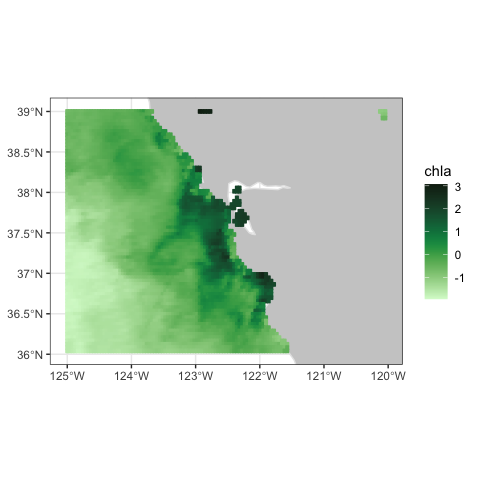### Crossing the date-line

The following is an `rxtracto_3D()` request that again uses the MUR dataset and crosses the date-line:

``````dataInfo <- rerddap::info('jplMURSST41mday')
parameter <- 'sst'
xcoord <- c(175, 185)
ycoord <- c(40, 50)
tcoord <- c('2019-03-16', '2019-03-16')
mur_dateline <- rxtracto_3D(dataInfo, parameter, xcoord = xcoord, ycoord = ycoord,
tcoord = tcoord)``````

## Plotting crossing the dateline

To make plots that cross the date-line, is is necessary to use the ‘world2’ continental outlines rather than the default. Due to some problems with that dataset, some regions must be removed in order to not get artificial lines.

``````xlim <- c(170, 190)
ylim <- c(40, 55)
remove <- c("UK:Great Britain", "France", "Spain", "Algeria", "Mali", "Burkina Faso", "Ghana", "Togo")
w <- map("world2Hires", xlim = xlim, ylim = ylim, fill = TRUE, plot = FALSE)
w <- map("mapdata::world2Hires", regions = w\$names[!(w\$names %in% remove)], plot = FALSE, fill = TRUE, ylim = ylim, xlim = xlim)``````

At present `plotBBox()` can plot across the date-line, but the x-axis labels get mixed up. Instead, the data can be plotted using `ggplot2`. A function `mapFrame()` is defined to help melt the data into a dataframe suitable for `ggplot2`, and then plotted using the outline defined above.

``````mapFrame <- function(longitude, latitude, my_data) {
my_data_name <- names(my_data)
temp_data <- drop(my_data[])
dims <- dim(temp_data)
temp_data <- array(temp_data, dims * dims)
my_frame <- expand.grid(x = longitude, y = latitude)
my_frame[my_data_name] <- temp_data
return(my_frame)
}
mur_frame <- mapFrame(mur_dateline\$longitude, mur_dateline\$latitude, mur_dateline['sst'])
mycolor <- cmocean::cmocean('thermal')(256)
myplot <- ggplot(data = mur_frame, aes(x = x, y = y, fill = sst)) +
geom_polygon(data = w, aes(x = long, y = lat, group = group), fill = "grey80") +     geom_tile(interpolate = FALSE) +
scale_fill_gradientn(colours = mycolor, na.value = NA) +
theme_bw() + ylab("latitude") + xlab("longitude") +
coord_fixed(1.3, xlim = xlim, ylim = ylim)
myplot``````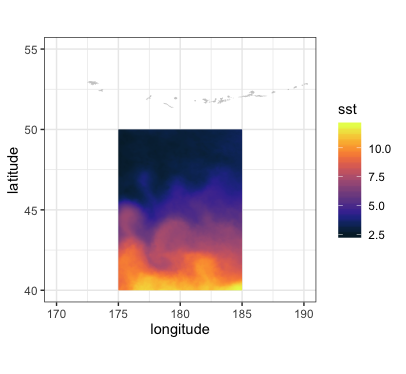## Using `rxtractogon`

The function `rxtractogon()` extracts a time-series of satellite data that are within a user supplied polygon. We repeat here two of the examples in the `xtractomatic` vignette. The first gets chlorophyll within the boundary points of the Monterey Bay National Marine Sanctuary, which are available in the `mbnms` dataset which are loaded with the `rerddapXtracto` package.

``````require("rerddapXtracto")
dataInfo <- rerddap::info('erdVH3chlamday')
parameter = 'chla'
tpos <- c("2014-09-01", "2014-10-01")
#tpos <-as.Date(tpos)
xpos <- mbnms\$Longitude
ypos <- mbnms\$Latitude
sanctchl <- rxtractogon(dataInfo, parameter = parameter, xcoord = xpos, ycoord = ypos,  tcoord = tpos)
str(sanctchl)
#> List of 6
#>  \$ chla       : num [1:50, 1:57, 1:2] NA NA NA NA NA NA NA NA NA NA ...
#>  \$ datasetname: chr "erdVH3chlamday"
#>  \$ longitude  : num [1:50(1d)] -123 -123 -123 -123 -123 ...
#>  \$ latitude   : num [1:57(1d)] 35.6 35.6 35.6 35.7 35.7 ...
#>  \$ altitude   : logi NA
#>  \$ time       : POSIXlt[1:2], format: "2014-09-15" "2014-10-15"
#>  - attr(*, "class")= chr [1:2] "list" "rxtracto3D"``````

The extract (see `str(sanctchl)`) contains two time periods of chlorophyll masked for data only in the sanctuary boundaries. This example shows how to pull out only a single time period to be used in `plotBBox()`.

``````require("ggplot2")
require("plotdap")
myFunc <- function(x) log(x)
sanctchl1 <- sanctchl
sanctchl1\$chla <- sanctchl1\$chla[, , 2]
sanctchl1\$time <- sanctchl1\$time
sanctchlPlot <- plotBBox(sanctchl1, plotColor = 'algae', myFunc = myFunc)
sanctchlPlot``````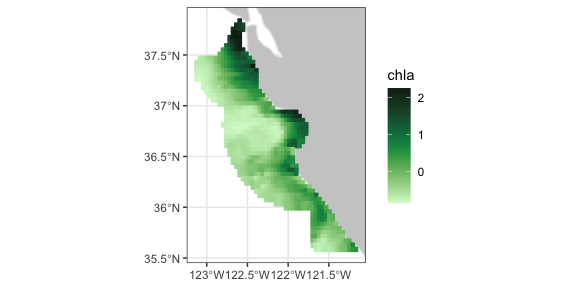This extract can be used to show the ability to animate the output through time:

``````require("gganimate")
require("ggplot2")
require("plotdap")
myFunc <- function(x) log(x)
sanctchlPlot <- plotBBox(sanctchl, plotColor = 'algae', myFunc = myFunc, time = identity, animate = TRUE)``````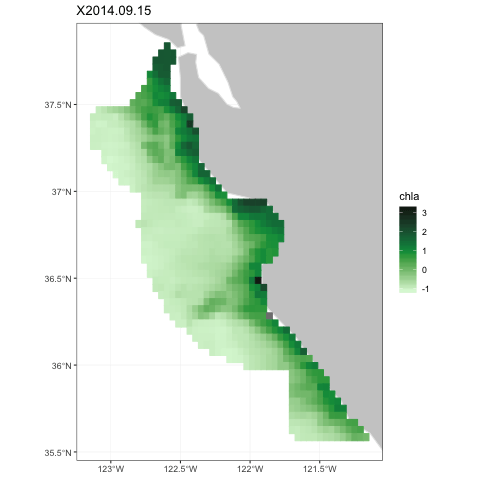Sanctuary Animation

The MBNMS is famous for containing the Monterey Canyon, which reaches depths of up to 3,600 m (11,800 ft) below surface level at its deepest. `rxtractogon()` can extract the bathymetry data for the MBNMS from the ETOPO dataset:

``````require("rerddap")
dataInfo <- rerddap::info('etopo180')
xpos <- mbnms\$Longitude
ypos <- mbnms\$Latitude
bathy <- rxtractogon(dataInfo, parameter = 'altitude', xcoord = xpos, ycoord = ypos)
str(bathy)
#> List of 6
#>  \$ depth      : num [1:123, 1:141, 1] NA NA NA NA NA NA NA NA NA NA ...
#>  \$ datasetname: chr "etopo180"
#>  \$ longitude  : num [1:123(1d)] -123 -123 -123 -123 -123 ...
#>  \$ latitude   : num [1:141(1d)] 35.5 35.6 35.6 35.6 35.6 ...
#>  \$ altitude   : logi NA
#>  \$ time       : logi NA
#>  - attr(*, "class")= chr [1:2] "list" "rxtracto3D"``````

Mapping the data to show the canyon:

``````require("ggplot2")
require("mapdata")
myFunc = function(x) -x
bathyPlot <- suppressMessages((plotBBox(bathy, plotColor = 'dense', myFunc = myFunc, name = 'Depth')))
bathyPlot``````